### Chaozhi Zheng, Otso Ovaskainen, Marjo Saastamoinen, and Ilkka Hanski. 2007. Age-dependent survival analyzed with Bayesian models of mark–recapture data. Ecology 88:1970–1976.

Appendix E. Bayesian regressions of individual deviations against traits.

The full posterior distribution of the model given by Eq. (3) is given by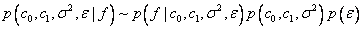,

where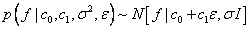with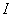is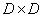identity matrix and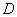is the dimension of vector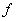, and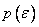is the marginal posterior distribution of the individual deviations in capture probabilities, which is obtained from the main model, and used here as a prior distribution. For the parameters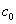,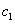, and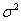we used a non-informative prior distribution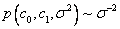. The full conditional posterior distributions used in the Gibbs sampling are given as follows.

1. The full conditional posterior distribution of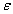is given by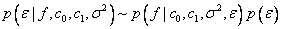.

The’s were sampled using the Metropolis-Hastings algorithm with proposal distribution.

2. The full conditional posterior distribution of the parameters,, andis given by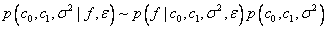.

These parameters were sampled using a standard procedure (Gelman et al. 2004), which involves two steps. (i)is sampled from the marginal posterior distribution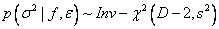, and (ii)andare sampled from the full conditional posterior distribution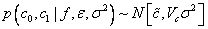. Here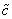,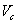, and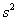are given by.

LITERATURE CITED

Gelman, A., J. B. Carlin, H. S. Stern, and D. B. Rubin. 2004. Bayesian data analysis. Chapman and Hall/CRC.

[Back to E088-117]